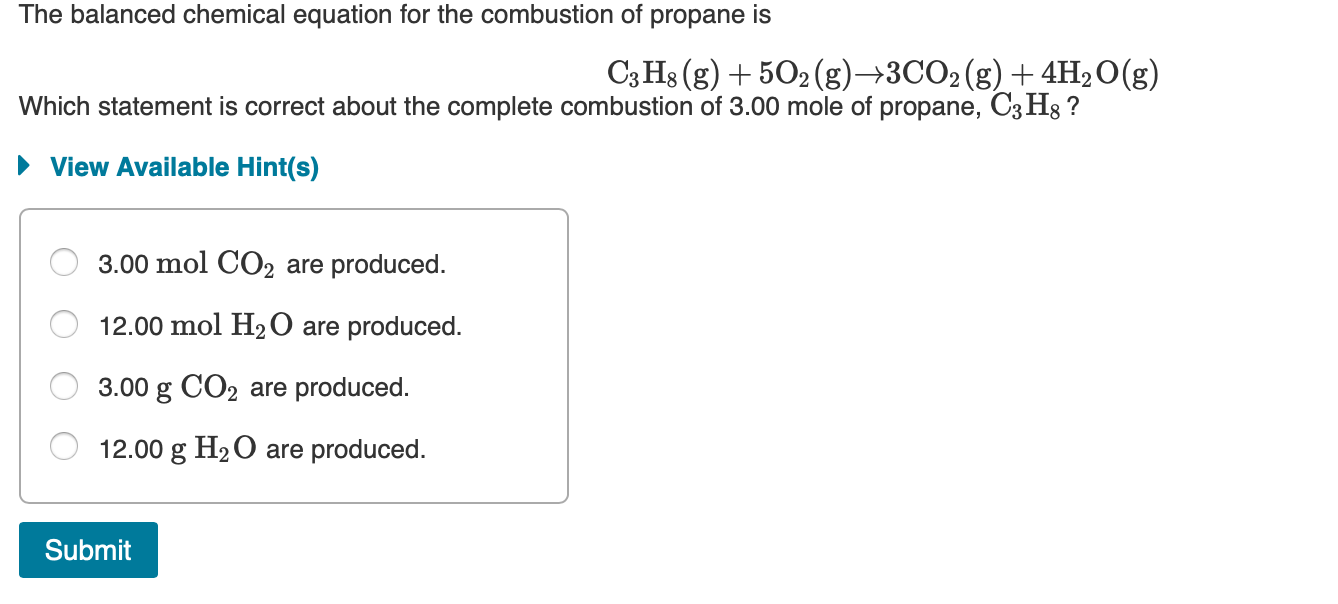Question

How molecules of CO2 (g) are produced when 0.75 moles of propane are burned? Chemical reaction: C3H8 + 5O2 à 3CO2 + 4H2O

moles C3H8 consumed = 0.75 mol

moles CO2 produced = (moles C3H8 consumed) * (mole ratio of CO2 to C3H8)

moles CO2 produced = (0.75 mol) * (3 mol CO2 / 1 mol C3H8)

moles CO2 produced = (0.75 mol) * (3)

moles CO2 produced = 2.25 mol

molecules CO2 produced = (moles CO2 produced) * (Avogadro's number)

molecules CO2 produced = (2.25 mol) * (6.022 x 1023 molecules/mol)

molecules CO2 produced = 1.355 x 1024 molecules

molecules CO2 produced = 1.4 x 1024 molecules        (correct number of significant figures)

#### Earn Coins

Coins can be redeemed for fabulous gifts.

Similar Homework Help Questions
• ### If you start with #7.0# moles of propane and #7.0# moles of oxygen gas what is the percent yield if #4.0# moles of carbon dioxide are produced?

Consider the reaction below. If you start with 7.0 moles of C3H8 (propane) and 7.0 moles of O2, _ is the percent yield if 4.0 moles of carbon dioxide is produced. C3H8(g) + 5O2(g) --> 3CO2(g) + 4H2O(g)

• ### The combustion of propane (C3H8) produces CO2 and H2O: C3H8 (g) + 5O2 (g) → 3CO2...

The combustion of propane (C3H8) produces CO2 and H2O: C3H8 (g) + 5O2 (g) → 3CO2 (g) + 4H2O (g) The reaction of 7.5 mol of O2 with 1.4 mol of C3H8 will produce ________ mol of CO2. Group of answer choices.

• ### The balanced chemical equation for the combustion of propane is C3H8(g) + 5O2(g) +3CO2(g) + 4H2O(g)...The balanced chemical equation for the combustion of propane is C3H8(g) + 5O2(g) +3CO2(g) + 4H2O(g) Which statement is correct about the complete combustion of 3.00 mole of propane, C3Hg ? ► View Available Hint(s) O 3.00 mol CO2 are produced. O 12.00 mol H2O are produced. O 3.00 g CO2 are produced. O 12.00 g H2O are produced. Submit

• ### Given the following equation, C3H8 (g) + 5O2 (g) -> 3CO2 (g) + 4H2O (g); H comb = -2219 kJ/mole A) how much heat will be released when 10 g of propane is burned

Given the following equation, C3H8 (g) + 5O2 (g) -> 3CO2 (g) + 4H2O (g); H comb = -2219 kJ/mole A) how much heat will be released when 10 g of propane is burned? B) how much heat will be released when 10 g of water (H2O) is formed? Please show all work.

• ### ( a) In the complete combustion of propane, how many moles of H2O(l) are produced per mole of CO2(g)? (b) A 1.638-g sample of propane is burned in excess oxygen. What are the theoretical yields (in grams) of CO2(g) and H2O(l) expected from the reaction

C3H8(g)+5O2(g)→ 3CO2(g)+4H2O(l)

• ### The combustion of propane, C3H8, occurs via the reaction C3H8(g)+5O2(g)→3CO2(g)+4H2O(g) with heat of formation values given...

The combustion of propane, C3H8, occurs via the reaction C3H8(g)+5O2(g)→3CO2(g)+4H2O(g) with heat of formation values given by the following table: Substance ΔH∘f (kJ/mol) C3H8 (g)=  -104.7 CO2(g)= −393.5 H2O(g)= −241.8 Calculate the enthalpy for the combustion of 1 mole of propane.

• ### 2. A normal dose of ibuprofen is 200. mg. How many ibuprofen molecules are there in...

2. A normal dose of ibuprofen is 200. mg. How many ibuprofen molecules are there in a dose of ibuprofen? The molecular formula of ibuprofen is C13H18O2. (Hint: grams to mol and mol to molecules) 3-Propane fuel is used in BBQs. Use the following reaction to determine how many moles of carbon dioxide gas are produced from burning of 6 moles of propane gas. C3H8(g) + 5O2(g) ®  4H2O(g) + 3CO2(g) 4- A. If a person inhales 51 moles of oxygen,...

• ### Consider the combustion of propane: C3H8 (g) + 5O2 (g) → 3CO2 (g) + 4H2O(l) ΔH...

Consider the combustion of propane: C3H8 (g) + 5O2 (g) → 3CO2 (g) + 4H2O(l) ΔH = –2221 kJ Assume that all of the heat comes from the combustion of propane. Calculate ΔH in which 5.00 g of propane is burned in excess oxygen at constant pressure.

• ### ﻿Propane (C3H8)burns according to the following balanced equation: C3H8(g)+5O2(g)→3CO2(g)+4H2O(g) Calculate ΔH∘rxnΔ for this reaction using standard...

﻿Propane (C3H8)burns according to the following balanced equation: C3H8(g)+5O2(g)→3CO2(g)+4H2O(g) Calculate ΔH∘rxnΔ for this reaction using standard enthalpies of formation. (The standard enthalpy of formation of gaseous propane is -103.9 kJ/molkJ/mol.) Express the enthalpy in kilojoules to four significant figures.

• ### The propane fuel (C3H8) used in gas barbeques burns according to this thermochemical equation. C3H8(g)+5O2(g)→3CO2(g)+4H2O(g)ΔH∘rxn=−2044kJ If...

The propane fuel (C3H8) used in gas barbeques burns according to this thermochemical equation. C3H8(g)+5O2(g)→3CO2(g)+4H2O(g)ΔH∘rxn=−2044kJ If a pork roast must absorb 1.7×103 kJ to fully cook, and if only 15 % of the heat produced by the barbeque is actually absorbed by the roast, what mass of CO2 is emitted into the atmosphere during the grilling of the pork roast?